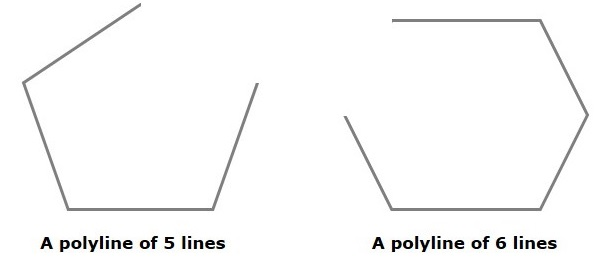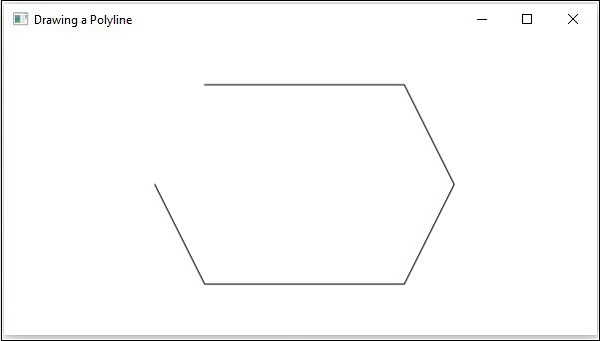# JavaFX - 2D Shapes Polyline

A Polyline is same as a polygon except that a polyline is not closed in the end. Or, continuous line composed of one or more line segments.

In short, we can say a polygon is an open figure formed by coplanar line segments.n JavaFX, a Polyline is represented by a class named Polygon. This class belongs to the package javafx.scene.shape..

By instantiating this class, you can create polyline node in JavaFX. You need to pass the x, y coordinates of the points by which the polyline should be defined in the form of a double array.

You can pass the double array as a parameter of the constructor of this class as shown below −

```Polyline polyline = new Polyline(doubleArray);
```

Or, by using the getPoints() method as follows −

```polyline.getPoints().addAll(new Double[]{List of XY coordinates separated by commas });
```

## Steps to Draw Polyline

To Draw a Polyline in JavaFX, follow the steps given below.

### Step 1: Creating a Class

Create a Java class and inherit the Application class of the package javafx.application and implement the start() method of this class as follows.

```public class ClassName extends Application {
@Override
public void start(Stage primaryStage) throws Exception {
}
}
```

### Step 2: Creating a Polyline

You can create a line in JavaFX by instantiating the class named Line which belongs to a package javafx.scene.shape. You can instantiate this class as follows.

```//Creating an object of the class Polyline
Polyline polyline = new Polyline();
```

### Step 3: Setting Properties to the Polyline

Specify a double array holding the XY coordinates of the points of the required polyline (hexagon in this example) separated by commas. You can do this by using the getPoints() method of the Polyline class as shown in the following code block.

```//Adding coordinates to the hexagon
200.0, 50.0,
400.0, 50.0,
450.0, 150.0,
400.0, 250.0,
200.0, 250.0,
150.0, 150.0,
});
```

### Step 4: Creating a Group Object

In the start() method create a group object by instantiating the class named Group, which belongs to the package javafx.scene.

Pass the Polyline (node) object, created in the previous step, as a parameter to the constructor of the Group class, in order to add it to the group as follows −

```Group root = new Group(polyline);
```

### Step 5: Creating a Scene Object

Create a Scene by instantiating the class named Scene which belongs to the package javafx.scene. To this class pass the Group object (root) that was created in the previous step.

In addition to the root object, you can also pass two double parameters representing height and width of the screen along with the object of the Group class as follows.

```Scene scene = new Scene(group ,600, 300);
```

### Step 6: Setting the Title of the Stage

You can set the title to the stage using the setTitle() method of the Stage class. The primaryStage is a Stage object which is passed to the start method of the scene class as a parameter.

Using the primaryStage object, set the title of the scene as Sample Application as follows.

```primaryStage.setTitle("Sample Application");
```

### Step 7: Adding Scene to the Stage

You can add a Scene object to the stage using the method setScene() of the class named Stage. Add the Scene object prepared in the previous steps using the following method.

```primaryStage.setScene(scene);
```

### Step 8: Displaying the Contents of the Stage

Display the contents of the scene using the method named show() of the Stage class as follows.

```primaryStage.show();
```

### Step 9: Launching the Application

Launch the JavaFX application by calling the static method launch() of the Application class from the main method as follows.

```public static void main(String args[]){
launch(args);
}
```

### Example

Following is a program which generates a polyline using JavaFX. Save this code in a file with the name PolylineExample.java.

```import javafx.application.Application;
import javafx.scene.Group;
import javafx.scene.Scene;
import javafx.stage.Stage;
import javafx.scene.shape.Polyline

public class PolylineExample extends Application {
@Override
public void start(Stage stage) {
//Creating a polyline
Polyline polyline = new Polyline();

200.0, 50.0,
400.0, 50.0,
450.0, 150.0,
400.0, 250.0,
200.0, 250.0,
150.0, 150.0,
});

//Creating a Group object
Group root = new Group(polyline);

//Creating a scene object
Scene scene = new Scene(root, 600, 300);

//Setting title to the Stage
stage.setTitle("Drawing a Polyline");

stage.setScene(scene);

//Displaying the contents of the stage
stage.show();
}
public static void main(String args[]){
launch(args);
}
}
```

Compile and execute the saved java file from the command prompt using the following commands.

```javac PolylineExample.java
java PolylineExample
```

On executing, the above program generates a JavaFX window displaying a polyline as shown below.javafx_2d_shapes.htm